Question

# At 500 ∘ C , cyclopropane ( C 3 H 6 ) rearranges to propene (...

At 500 ∘ C , cyclopropane ( C 3 H 6 ) rearranges to propene ( CH 3 −CH= CH 2 ). The reaction is first order, and the rate constant is 6.7× 10 −4 s −1 . If the initial concentration of C 3 H 6 is 5.50×10−2 M . Part A Part complete What is the half-life (in minutes) of this reaction? Express your answer using two significant figures. t 1/2 = 17 min SubmitPrevious Answers Correct Part B How many minutes will it take for the concentration of cyclopropane to drop to 12.5% of its initial value? Express your answer using two significant figures. t t = 69 min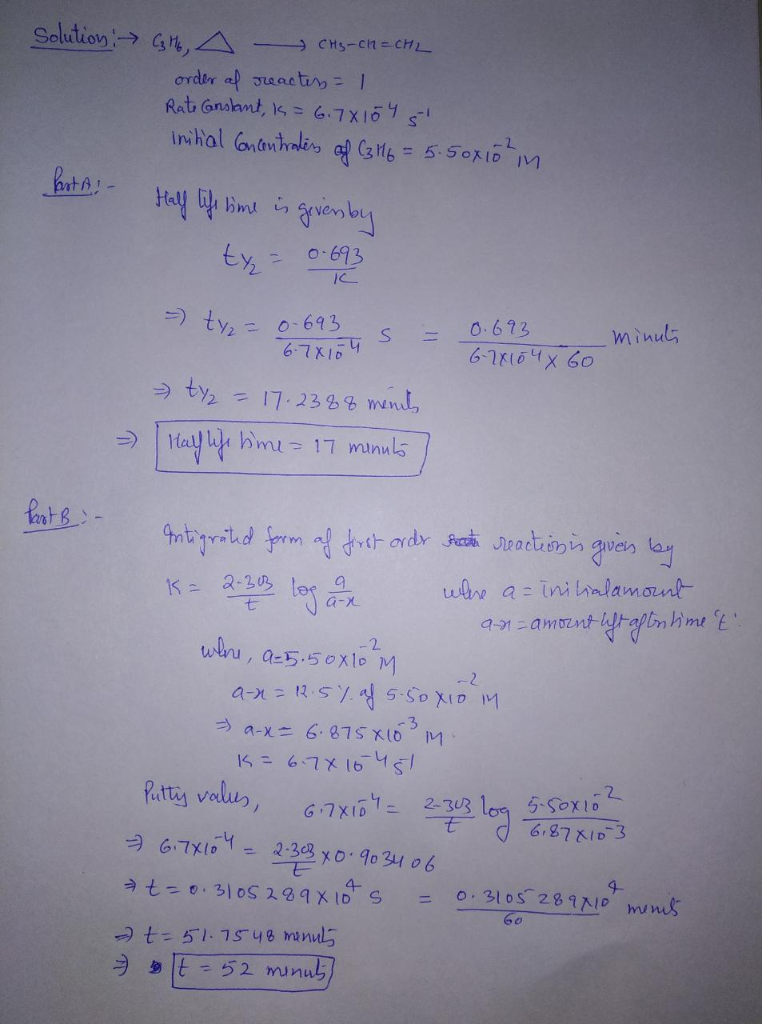#### Earn Coins

Coins can be redeemed for fabulous gifts.

Similar Homework Help Questions
• ### At 500 ∘C, cyclopropane (C3H6) rearranges to propene (CH3−CH=CH2). The reaction is first order, and the...

At 500 ∘C, cyclopropane (C3H6) rearranges to propene (CH3−CH=CH2). The reaction is first order, and the rate constant is 6.7×10−4s−1. If the initial concentration of C3H6 is 5.50×10−2 M . What is the molarity of C3H6 after 30 min ? How many minutes does it take for the C3H6 concentration to drop to 1.00×10−2 M ? How many minutes does it take for 25% of the C3H6 to react? Express all the answers in two significant figures.

• ### Cyclopropane rearranges to form propene in the gas phase. Part A If the initial cyclopropane concetration...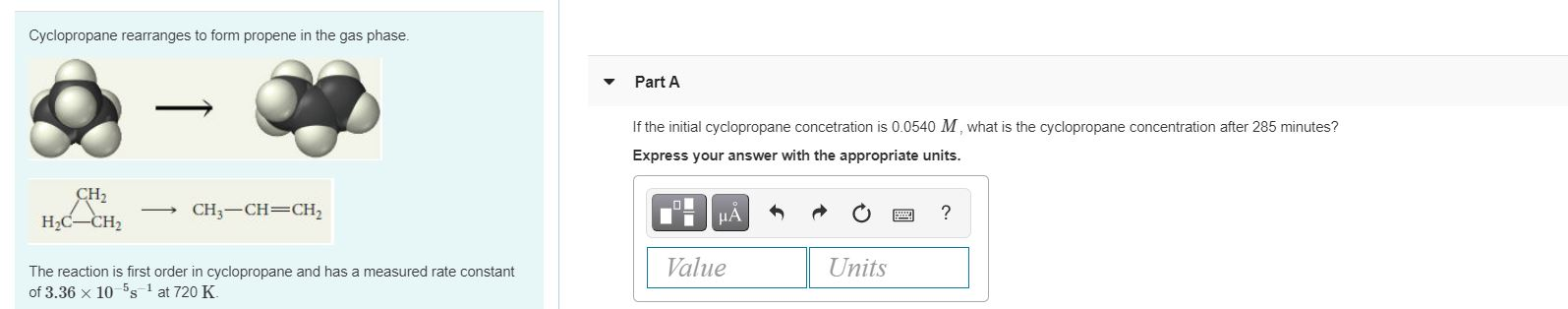Cyclopropane rearranges to form propene in the gas phase. Part A If the initial cyclopropane concetration is 0.0540 M what is the cyclopropane concentration after 285 minutes? Express your answer with the appropriate units. CH2 HC-CH2 CH3-CH=CH2 μΑ ? Value Units The reaction is first order in cyclopropane and has a measured rate constant of 3.36 x 10-5s-1 at 720 K

• ### At 500 oC cyclopropane (C3H6) rearranges to propene (CH3CHCH2) as shown by C3H6(g) -->CH3CHCH2(g). The reaction...

At 500 oC cyclopropane (C3H6) rearranges to propene (CH3CHCH2) as shown by C3H6(g) -->CH3CHCH2(g). The reaction is first-order with a half-life of 17 minutes. How long will it take for the concentration of C3H6 to drop to 12.5% of its initial value at 500 oC?

• ### 2. At 500 °C, cyclopropane (C3H6) rearranges to propene (CH3CH=CH2): C3H6 (g) → CH3CH=CH2 (8) The reaction is first...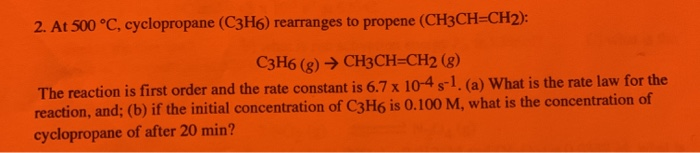2. At 500 °C, cyclopropane (C3H6) rearranges to propene (CH3CH=CH2): C3H6 (g) → CH3CH=CH2 (8) The reaction is first order and the rate constant is 6.7 x 10-4 5-1. (a) What is the rate law for the reaction, and; (b) if the initial concentration of C3H6 is 0.100 M, what is the concentration of cyclopropane of after 20 min?

• ### Cyclopropane rearranges to form propene in the gas phase. Constant Part A If the initial cyclopropane...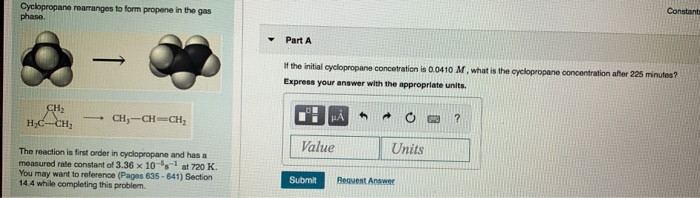Cyclopropane rearranges to form propene in the gas phase. Constant Part A If the initial cyclopropane concetration is 0.0410 M, what is the cyclopropane concentration after 225 minutes? Express your answer with the appropriate units. CH HC-CH CH ---CH=CH, HA ? Value Units The reaction is first order in cyclopropane and has a measured rate constant of 3.36 x 10's-1 at 720 K. You may want to reference (Pages 635 - 641) Section 14.4 while completing this problem Submit Request...

• ### Cyclopropane rearranges to form propene in the gas phase. Constants Periodic Table Part A - If...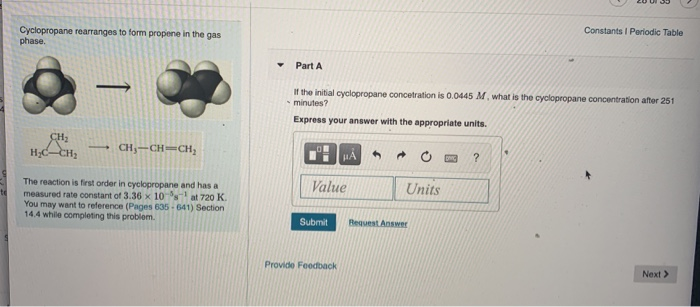Cyclopropane rearranges to form propene in the gas phase. Constants Periodic Table Part A - If the initial cyclopropane concetration is 0.0445 M. what is the cyclopropane concentration after 251 minutes? Express your answer with the appropriate units. CH, H₂CH₂ CH3-CH=CH μΑ ? Value Units The reaction is first order in cyclopropane and has a measured rate constant of 3.36 x 10-ºsat 720 K. You may want to reference (Pages 635-641) Section 14.4 while completing this problem. Submit Request Answer...

• ### Constants Periodic Table Cyclopropane rearranges to form propene in the gas phase Part A If the...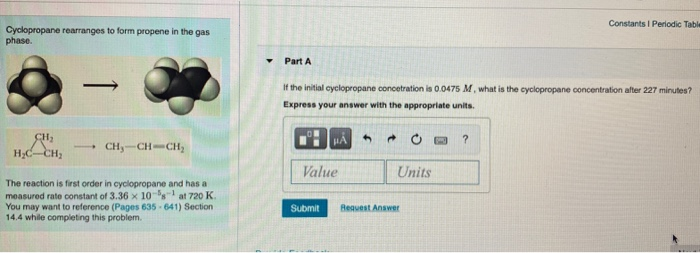Constants Periodic Table Cyclopropane rearranges to form propene in the gas phase Part A If the initial cyclopropane concetration is 0.0475 M. what is the cyclopropane concentration after 227 minutes? Express your answer with the appropriate units. CH MÅ ? нс-Сн, CHY-CH-CH: Value Units The reaction is first order in cyclopropane and has a measured rate constant of 3.36 x 10-8-- at 720 K You may want to reference (Pages 635.641) Section 14.4 while completing this problem Submit Request Answer

• ### Constants I Periodic Table Cyclopropane rearranges to form propene in the gas phase Part A If...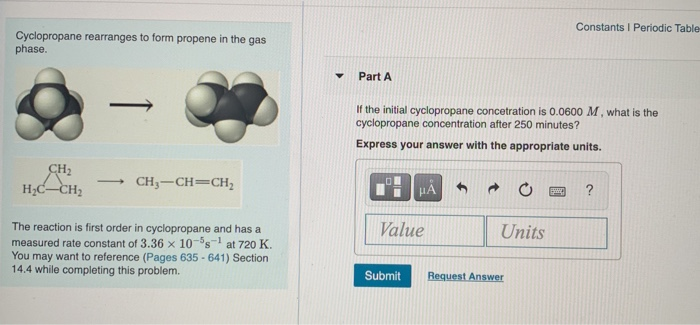Constants I Periodic Table Cyclopropane rearranges to form propene in the gas phase Part A If the initial cyclopropane concetration is 0.0600 M , what is the cyclopropane concentration after 250 minutes? Express your answer with the appropriate units. CH2 HC-CH2 CH3 -CH=CH НА ? Value Units The reaction is first order in cyclopropane and has a measured rate constant of 3.36 x 10-5s-1 at 720 K. You may want to reference (Pages 635 - 641) Section 14.4 while completing...

• ### Cyclopropane was used in a mixture with oxygen as an anesthetic. When heated, cyclopropane rearranges to...

Cyclopropane was used in a mixture with oxygen as an anesthetic. When heated, cyclopropane rearranges to propene in a first-order process. If the initial concentration is 0.050 mol/L how much time (in hours) must elapse for its concentration to drop to 0.010 mol/L ? k is 2.42 h-1

• ### Need ALL answered! 1. In the hydrogenation of ethylene using a nickel catalyst, the initial concentration...

Need ALL answered! 1. In the hydrogenation of ethylene using a nickel catalyst, the initial concentration of ethylene is 1.80 mol⋅L−1 and its rate constant (k) is 0.0016 mol⋅L−1⋅s−1 . Determine the rate of reaction if it follows a zero-order reaction mechanism. Express your answer to two significant figures and include the appropriate units. 2. The reaction 2X →products is a second-order reaction, and the rate constant is 8.8 × 10−3 1/M·s. If the initial concentration of X is 3.00...